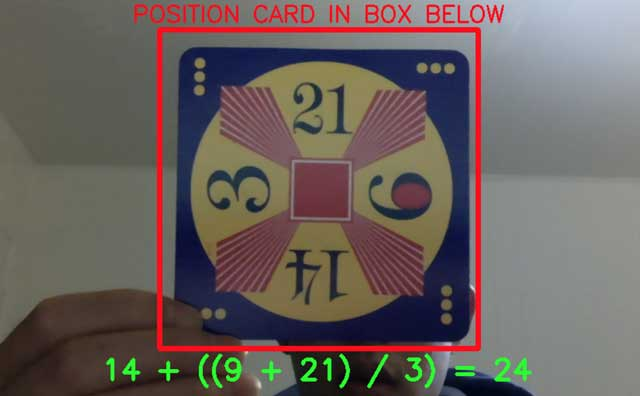# Dominick Lim

home - projects - github

### 24 SolverThe 24 Game is a card game in which the objective is to find a way to manipulate four integers so that the end result is 24. For example, for the card with the numbers 3, 9, 14, and 21, a possible solution is 14 + ((9 + 21) / 3) = 24.

I built a Python script that detects the numbers on a card and then presents a possible solution. I wrote the computer vision algorithm to detect the numbers on the card, and the algorithm to find a solution given those numbers.

Built with: Python, Numpy, OpenCV.